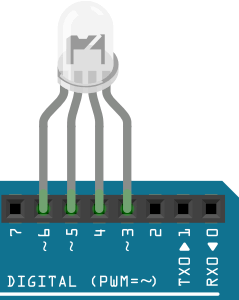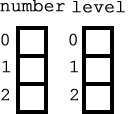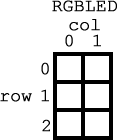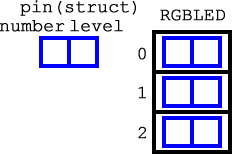Data Modeling 1 ExercisesWe have placed an emphasis on the writing of great code. Since code is typically developed to manipulate data, you also need to start thinking about designing efficient and robust data structures that lend themselves to the pursuit of great coding practices.

Consider the 4-pin CC RGBLED that is in your toolkit and the code we could write to exercise its performance. To keep the hardware side of this investigation as simple as possible, the leads of the pins could be inserted as shown to the right. In this way, we have PWM pins (3,5, & 6) )to drive each of the anode leads and a standard digital pin (4) for the single common cathode.

Code control over the device would need to reference the pin number (3, 5, or 6) and its state (0-255). The most elementary data model would be to use 6, uint8_t variables. This simplistic strategy does not scale well for more LEDs and provides the loosest of bindings (by identifier only as is pinRed and stateRed) so we'll reject it.

Create the Arduino Project, RGBModel, and let's explore some options.

Model 1 (Poor). Parallel ArraysWe could declare two separate arrays (referred to as parallel arrays) as in,

```uint8_t number[] = {3,5,6};
uint8_t level[] = {16, 2, 255};
```
In this case, the pin number and its level are only loosely bound by sharing the same index within each array. This is a little better than the 6-variable suggestion above. Implement this data design and develop code to run this continuously with 1 second intervals between transitions.

Model 2 (Better). Two-Dimensional Array (Matrix)A tighter binding results from having a two-dimensional array (matrix) in which elements within each horizontal row are considered related. The first column is interpreted as the pin number and the second column represents the level. The data structure is declared and populated as follows,

`uint8_t RGBLED[][] = {{3,16), {5,2}, {6,255} };`

This model provides a tight binding (one single variable) but suffers from the user having to remember what each column represents. Modify the RGBModel sketch by commenting out the previous code and implementing a matrix-based data model.

Model 3 (Best of this group-IMO). Array of Structs

The concept of a in C predates the notion of an object of a class that didn't arrive on the programming scene until the 1980s. Hierarchical combinations of structs and arrays can lead to some terrific data modeling options.The blue bonded pair of fields to the left is meant to convey a struct variable by the name of pin, defining two, tightly-bound fields, number and state. The data model, RGBLED can be defined as an array of struct, defined and initially populated as follows,

```struct pin {
uint8_t number;
uint8_t level;
};
pin RGBLED[] = {{3, 16}, {5, 2}, {6, 255}};
```
Modify the RGBModel sketch by commenting out the previous code and implementing an array of struct data model. Once your code is complete you may appreciate the benefit of named fields that enhance your code's readability.